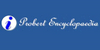### OperandIn mathematics, an operand is the object of a mathematical operation, a quantity on which an operation is performed. ==Example == In the above example, `+` is the symbol for the operation called addition. The operand `3` is one of the inputs (quantities) followed by the addition operator, and the operand `6` is the other input necessary for ...
Found on http://en.wikipedia.org/wiki/Operand

### Operand• (n.) The symbol, quantity, or thing upon which a mathematical operation is performed; -- called also faciend.
Found on http://thinkexist.com/dictionary/meaning/operand/

### operandnoun a quantity upon which a mathematical operation is performed
Found on https://www.encyclo.co.uk/local/20974

### OperandOp'er·and noun [ From neuter of Latin operandus , gerundive of operari . See Operate .] (Math.) The symbol, quantity, or thing upon which a mathematical operation is performed; -- called also faciend .
Found on http://www.encyclo.co.uk/webster/O/23

### OperandIn mathematics, an operand is the symbol, quantity, or thing upon which a mathematical operation is performed.
Found on http://www.probertencyclopaedia.com/browse/GO.HTM

### operand[n] - a quantity upon which a mathematical operation is performed
Found on http://www.webdictionary.co.uk/definition.php?query=operand
No exact match found